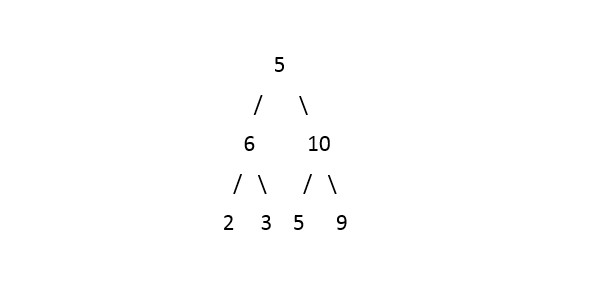# Largest Number in Binary Tree

Posted: 9 Oct, 2020
Difficulty: Moderate

## PROBLEM STATEMENT

#### Your task is to find the largest number that could be formed by concatenating all its nodes values.

##### For example:
``````For the given binary tree:
````````````Output: 96553210

Explanation: After concatenating all the numbers in the above binary tree this is the largest number that can be formed.
``````
##### Input Format:
``````The first line of input contains a single integer 'T', representing the number of test cases.

The only line of each test case contains elements in the level order form. The line consists of values of nodes separated by a single space. In case a node is null, we take -1 in its place.

Example:
The input for the tree depicted in the below image would be:
1 2 3 4 -1 5 6 -1 7 -1 -1 -1 -1 -1 -1
``````#### Explanation :

``````Level 1 :
The root node of the tree is 1

Level 2 :
Left child of 1 = 2
Right child of 1 = 3

Level 3 :
Left child of 2 = 4
Right child of 2 = null (-1)
Left child of 3 = 5
Right child of 3 = 6

Level 4 :
Left child of 4 = null (-1)
Right child of 4 = 7
Left child of 5 = null (-1)
Right child of 5 = null (-1)
Left child of 6 = null (-1)
Right child of 6 = null (-1)

Level 5 :
Left child of 7 = null (-1)
Right child of 7 = null (-1)

1
2 3
4 -1 5 6
-1 7 -1 -1 -1 -1
-1 -1
``````
##### Note:
``````The first node that is not null (of the previous level) is treated as the parent of the first two nodes of the current level. The second not-null node (of the previous level) is treated as the parent node for the next two nodes of the current level and so on. The input ends when all nodes at the last level are null (-1).
``````
##### Output Format:
``````For each test case, print the integer that represents the largest number that could be formed by concatenating all its nodes given in a Binary Tree.
``````
##### Note:
``````You don't need to print anything, it has already been taken care of. Just implement the given function.
``````
##### Constraints:
``````1 <= T <= 5
0 <= N <= 10^4
0 <= data <= 10^3

Where 'N' denotes the total number of nodes and 'data' denotes the node value of the Binary Tree.

Time limit: 1 sec
``````Approach 1

A simple solution would be to store all the node values to the list/array by traversing the whole Binary Tree and the problem now is that given a list of non-negative integers, arrange them such that they form the largest number. To solve this problem, sort the array in descending order, but sorting the list/array does not work here.

The idea is to use any of the comparison-based sorting algorithms.

1. We will write our own custom comparator function for sorting.
2. For the two numbers ‘A’ and ‘B’. This custom function will not compare ‘A’ and ‘B’ with each other. But it compares ‘AB’ with ‘BA’ and the greater number will come first in sorted order.
3. Here ‘AB’ means the number is formed by appending ‘A’ to ‘B’  and vice versa for 'BA'.

For Example 'A' = 213 and 'B' = 75 then ‘AB’ = 21375 and ‘BA’ = 75213.To compare 'A' and 'B' our custom compare function will compare ‘AB’ and ‘BA’, Since ‘AB’ < ‘BA’ so, we will put ‘B’ first in the sorted list/array.